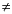Functions and CALL Routines

# DCLOSE Function

Closes a directory that was opened by the DOPEN function.
 Category: External Files

## Syntax

 DCLOSE(directory-id)

### Argument

directory-id

is a numeric variable that specifies the identifier that was assigned when the directory was opened by the DOPEN function.

DCLOSE returns 0 if the operation was successful,0 if it was not successful. The DCLOSE function closes a directory that was previously opened by the DOPEN function. DCLOSE also closes any open members.

Note:   All directories or members opened within a DATA step are closed automatically when the DATA step ends.### Example 1: Using DCLOSE to Close a Directory

This example opens the directory to which the fileref MYDIR has previously been assigned, returns the number of members, and then closes the directory:

```%macro memnum(filrf,path);
%let rc=%sysfunc(filename(filrf,&path));
%if %sysfunc(fileref(&filrf)) = 0 %then
%do;
/* Open the directory. */
%let did=%sysfunc(dopen(&filrf));
%put did=&did;
/* Get the member count. */
%let memcount=%sysfunc(dnum(&did));
%put &memcount members in &filrf.;
/* Close the directory. */
%let rc= %sysfunc(dclose(&did));
%end;
%else %put Invalid FILEREF;
%mend;
%memnum(MYDIR,physical-filename)```

### Example 2: Using DCLOSE within a DATA Step

This example uses the DCLOSE function within a DATA step:

```%let filrf=MYDIR;
data _null_;
rc=filename("&filrf","physical-filename");
if fileref("&filrf") = 0 then
do;
/* Open the directory. */
did=dopen("&filrf");
/* Get the member count. */
memcount=dnum(did);
put memcount "members in &filrf";
/* Close the directory. */
rc=dclose(did);
end;
else put "Invalid FILEREF";
run;```

 Functions:Previous Page | Next Page | Top of Page